### Cryptocurrency

Cryptonia prospered thanks to the new gold mine, the security provided by the dragon, and the convenience of the DragonBucks system.

This brought an influx of non-native Cryptonians to the area, who provided fresh energy and industry to the burgeoning city. It also drove the dishonest outsiders out of the town in search of easier prey, since DragonBucks made theft impossible for them.However, it also introduced a problem: while newcomers could receive DragonBucks, they couldn’t sign their own notes, as they weren’t born with the ability to cast a unique spell. The members of the newly formed Cryptonian Academy of Scholars gathered to come up with a solution.They figured that their best shot to integrate the non-magical denizens of Cryptonia into the new currency would be to use one of the closest things the rest of us have to magic: mathematics.

# Cryptographic Signatures

The Cryptonian scholars need to find mathematical replacements for the functions of DragonBucks. In particular, they need something to replace the dragon so that people can verify that a transaction is valid, and they need replacements for spells as personal identities.

To make it easier to talk about these replacements, we can give names to each part of the system.$^*$ Since a Cryptonian’s spellcasting ability is personal to them, it's called a $\tt secretKey.$ The visual spell effect is connected to this spellcasting ability but can be publicly shared, so it's a $\tt publicKey.$ A transaction note is a $\tt message,$ and the enchanted wax seal is a $\tt signature$ since it's used to prove who sent a message.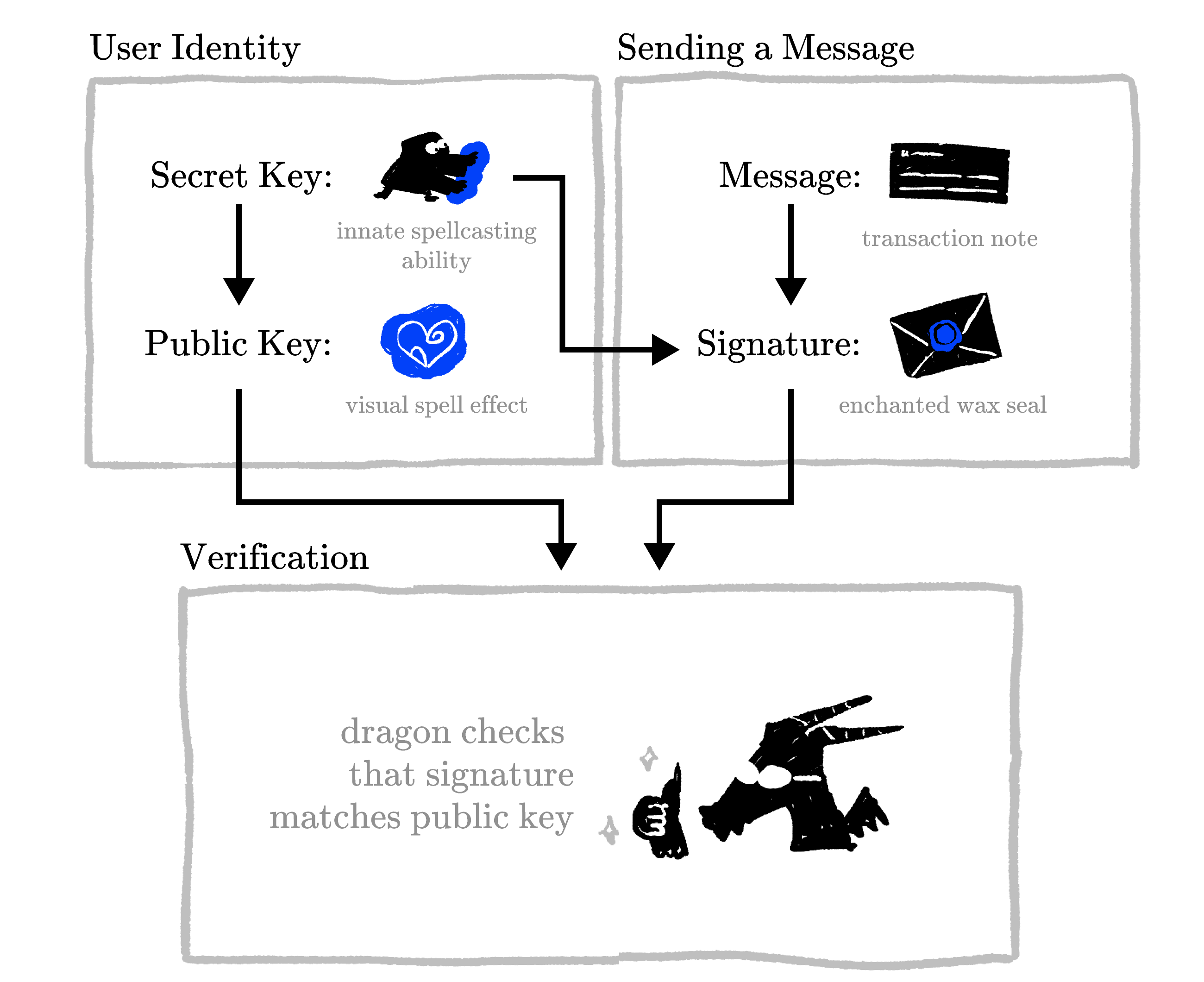The crucial step is being able to verify a $\tt signature$ while hiding the $\tt secretKey$ that generated it. In this quiz, you'll learn about a mathematical function that hides information and can help us towards this goal.

$^*$We're borrowing these names from public-key cryptography, but you don't need to be familiar with public-key cryptography to understand this quiz.

# Cryptographic Signatures

The Cryptonian scholars start by considering a very simple mathematical system that uses numbers in place of spells and transaction notes and also uses multiplication to move between each step. Here's how user identities and sending messages would work in this system:

1. Everyone picks a number $s$ for their $\tt secretKey$ which they don't reveal, but they share $5\times s$ as their $\tt publicKey$.
2. Each $\tt message$ is converted into a number $m$.*
3. The person sending the $\tt message$ produces a $\tt signature$ to prove they're the one who sent it by calculating $m \times s$.

For example, Alice has a $\tt publicKey$ of $35$ and wants to send the $\tt message$ $101$ (a real message would be much longer, but we've truncated it so that you don't need to find your calculator):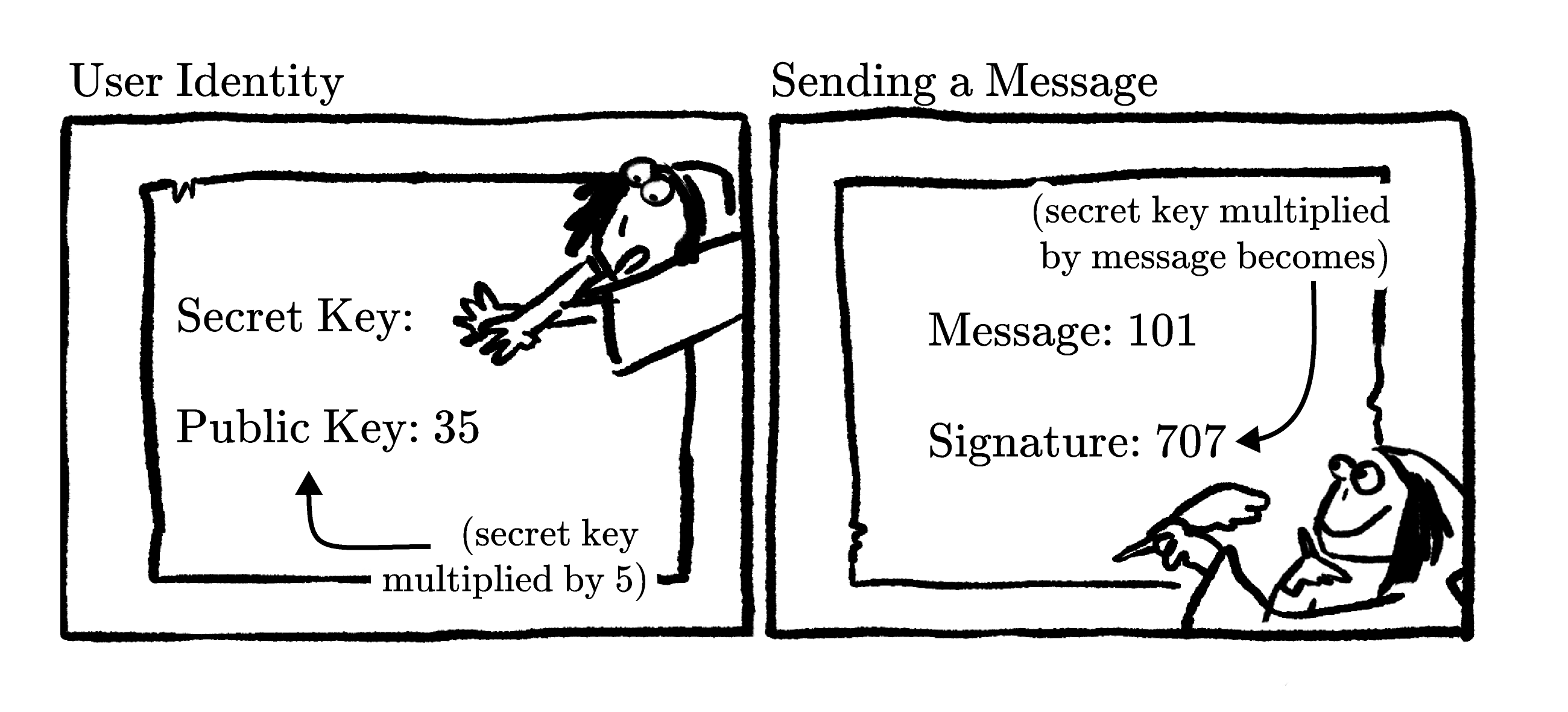The last piece of the system is that we need to be able to verify that Alice was the one to produce her $\tt signature$.

What equation must be true if Alice's $\tt signature$ of $707$ was calculated from the product of her $\tt secretKey$ and $\tt message$?

*An example of how to perform this conversion is examined later in the chapter. You'd need to be careful about the details of how to convert a message to a number when building a real system, but for now we can just trust that it's possible.

# Cryptographic Signatures

In the proposed system, we can verify a $\tt signature$ by checking that the following equation is true: $\texttt{signature}\times 5 = \texttt{publicKey}\times m,$ because if we substitute in the $\texttt{secretKey}\ s$ and $\texttt{message}\ m$ used to generate the $\tt signature$ and $\tt publicKey$, it produces $\overbrace{\left(m\times s\right)}^\texttt{signature}\times 5 = \overbrace{\left(5 \times s\right)}^\texttt{publicKey} \times m.$ The order of multiplication doesn't matter and both these expressions have the exact same factors $(5, m,$ and $s)$, so they'll be equal for a valid $\tt signature$.

When Alice $(\texttt{publicKey} = 35)$ sends the $\tt message$ $101$ with the $\tt signature$ $707,$ this will check out since $707 \times 5 = 35 \times 101.$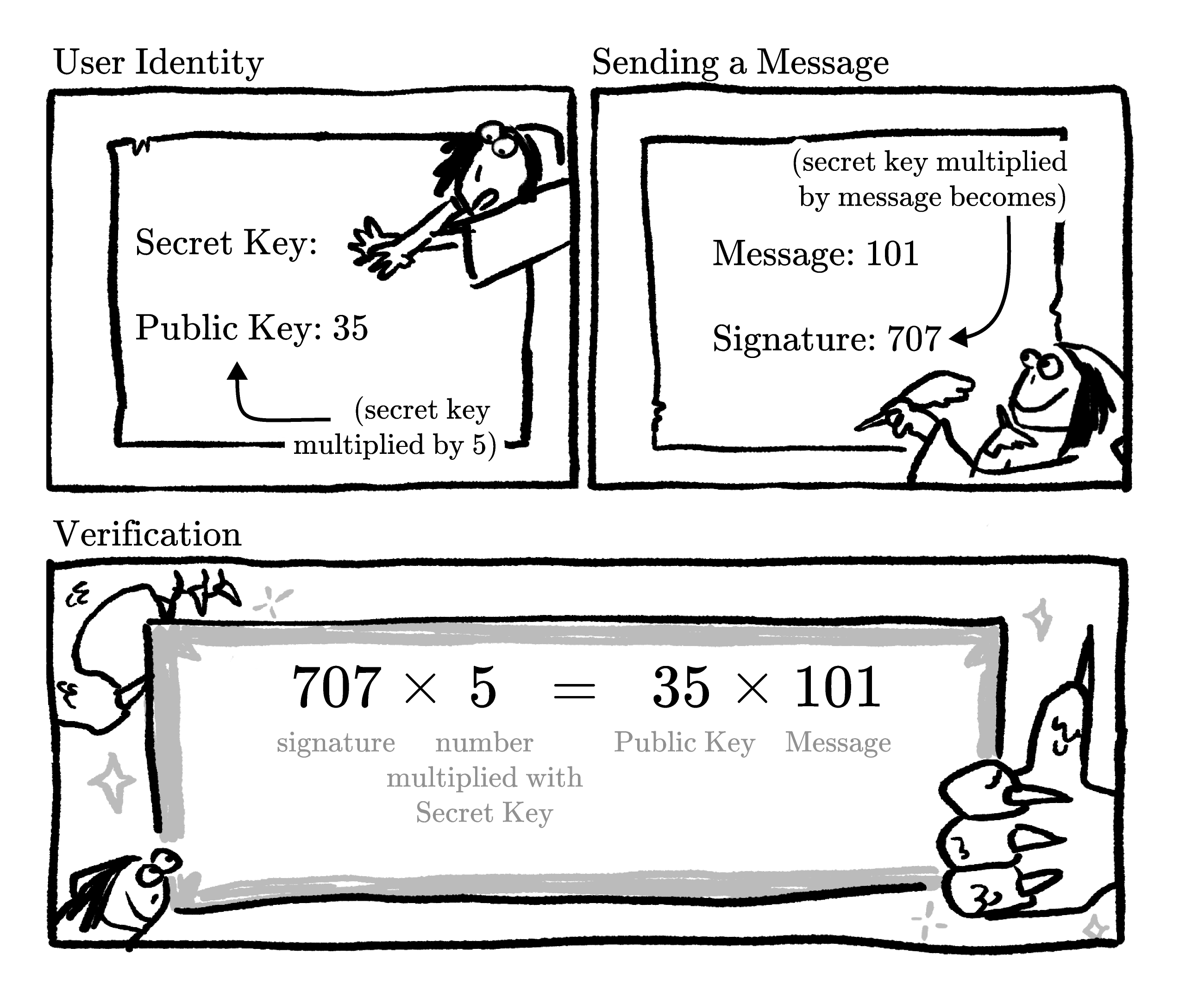But this belies the real problem with the system: it isn't secure. What is Alice's $\tt secretKey$?

# Cryptographic Signatures

Your $\tt signature$ needs to be something that only you can produce. The simple multiplication scheme doesn't work because anyone who understands the rules of the system can steal your $\tt secretKey$ just by dividing!

To replace DragonBucks, we need to be able to verify a $\tt signature$ without compromising the security of the $\tt secretKey$ associated with it.

Which of the following ways of calculating a $\tt publicKey$ would prevent you from immediately determining the exact value of the $\tt secretKey$ used to generate it?

Assume that the $\tt secretKey$ is always a positive integer.

# Cryptographic Signatures

Most ordinary functions don't hide their inputs very well: you can reverse addition with subtraction, division with multiplication, squaring with taking the square root, and so on.

Fortunately, there are some functions that can't be easily reversed. For example, if we share the remainder after dividing a $\tt secretKey$ by $17,$ it doesn't reveal the $\tt secretKey$: there are infinite possibilities. If the remainder of $s$ divided by $17$ is $5$, $s$ could be $5$ or $22$ or $39$ or $56$ or $\ldots$

The modulo operation $(\bmod{}$ for short$)$ divides by a number (the "modulus") and returns the remainder, so we can write the situation above as $(\text{remainder}) = s\bmod{17}.$

Consider $63 \bmod{17}:$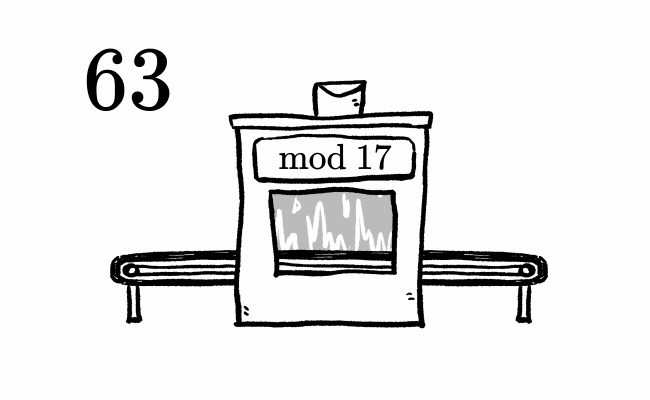We can view $63$ as its remainder plus some multiple of $17:$ $63 = 12 + 3 \times 17 = (\text{remainder}) + (\text{some number}) \times 17.$ $\bmod{\>17}$ keeps the remainder, but the multiples of $17$ are lost, so that part of the original number remains hidden.

If we can use $\bmod{}$ to verify a $\tt signature$ while hiding the $\tt secretKey$ that generated it, that will help us mathematize the DragonBucks system.

# Cryptographic Signatures

Taking the remainder of a lone number hides information about that number, so perhaps taking the remainder after multiplication will hide information about the factors that went into that multiplication.

This could make $\bmod{}$ especially helpful for hiding the $\tt secretKey$ used to generate a $\tt signature$.

If we multiplied the $\tt message$ by the $\tt secretKey$ and then took the remainder after dividing by $n:$ $(m\times s) \bmod{n},$ using this value as the $\tt signature$ instead of $m\times s$ might hide our $\tt secretKey$ better.

Alice is going to send the $\tt message$ $101$ and generates a $\tt signature$ by calculating $(m\times s) \bmod{17}$. If her $\tt signature$ is $12$, could we calculate Alice's $\tt secretKey$?

# Cryptographic Signatures

Using $(m \times s)\bmod{n}$ to generate a $\tt signature$ does a much better job of hiding the $\tt secretKey$ used to produce it — simple division will no longer reveal $s$.

Graphically, $m \times s$ is the area of a rectangle with side lengths $m$ and $s.$ If we apply $\bmod{\>n}$ to that area, any multiples of $n$ in it are lost, and it becomes much harder to find $m$ or $s$ from that remainder: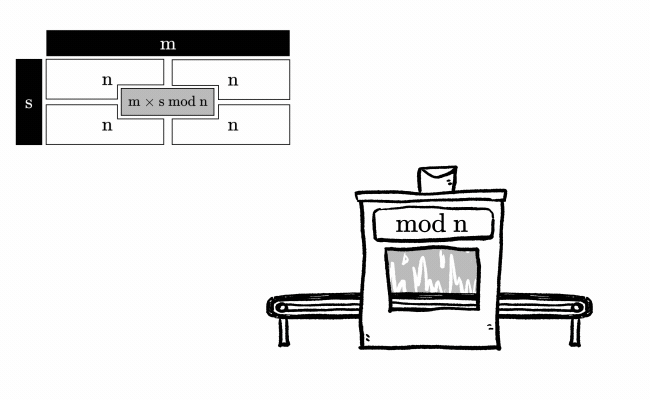This is because as long as $m \times s$ is bigger than $n,$ at least one multiple of $n$ will get thrown out when $\bmod{\>n}$ is applied. Losing any part of $m \times s$ means that dividing by $m$ will not recover $s.$

# Cryptographic Signatures

Instead of just dividing the $\tt signature$ to find $s$, a would-be impostor now has a lot more work ahead of them. The simplest approach they could use would be to try different values of $t$ until they found one such that \begin{aligned} (m\times t)\bmod{n} &= \texttt{signature} \\ &= (m\times s)\bmod{n}. \end{aligned} The value of $t$ satisfying this equation would be a candidate for $s$.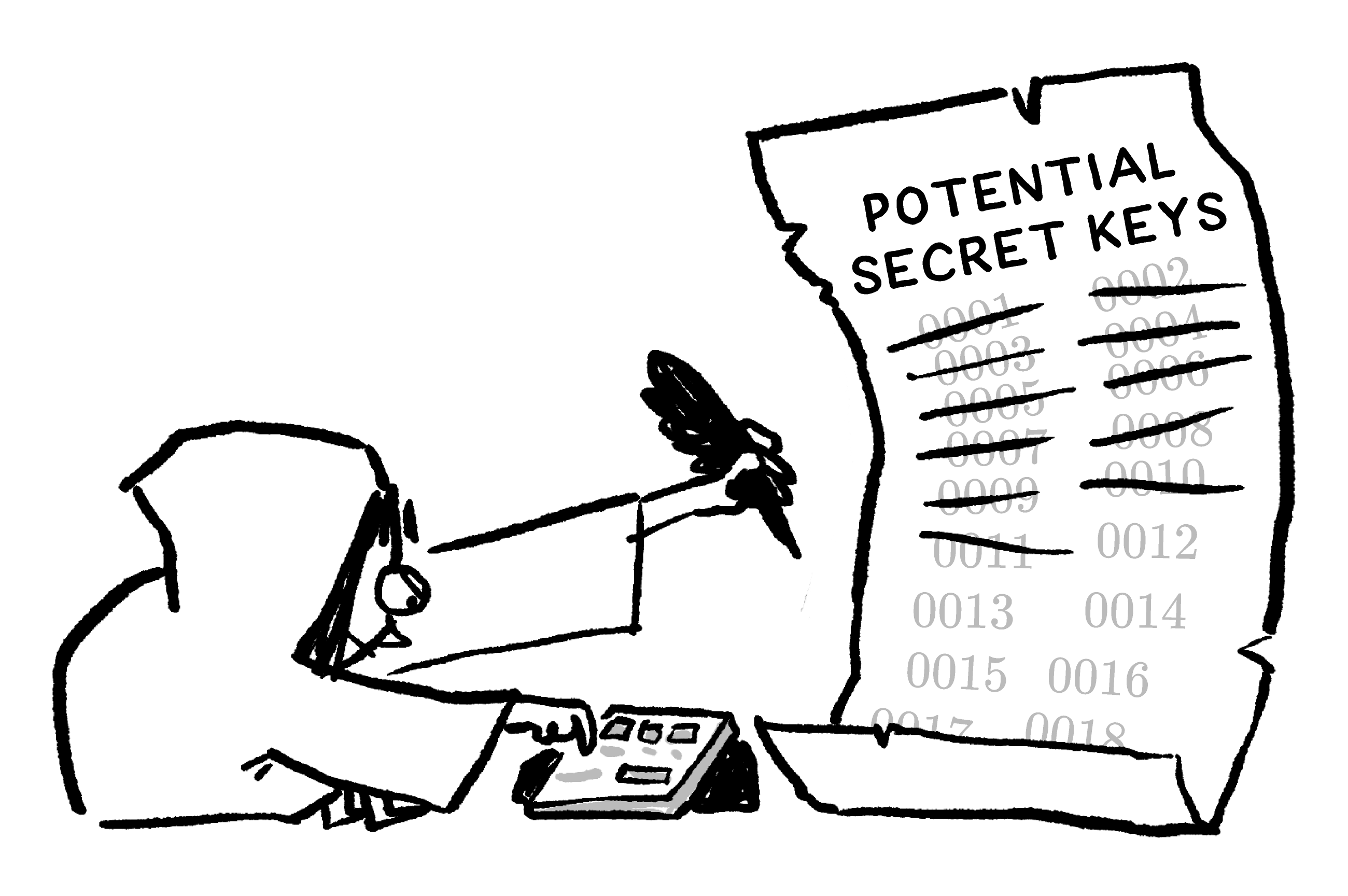But if we make the numbers big enough ($n$, in particular), then we can make this search take a long time and therefore keep $\tt secretKey$ safe. This approach lets someone publish a $\tt signature$ without revealing their $\tt secretKey,$ one of the key properties that will allow us to mathematize DragonBucks!

# Cryptographic Signatures

With $\bmod{}$ in our toolbox, we're ready to take another crack at the mathematization of DragonBucks. Supercharged with modular arithmetic, our naive multiplication scheme might not be so bad after all.

Since $\bmod{}$ allows us to hide inputs, we can integrate modular arithmetic into an updated version of the system. Here's how user identities and sending messages could work after the update:

1. Everyone agrees on a number $n$ to use as the modulus of the system.
2. Everyone picks a $\tt secretKey$ $s$ which they don't reveal, but they share $s\bmod{n}$ as their $\tt publicKey$.
3. Each $\tt message$ is converted into a number $m$.
4. The $\tt signature$ for a $\tt message$ $m$ is $(m \times s)\bmod{n}$.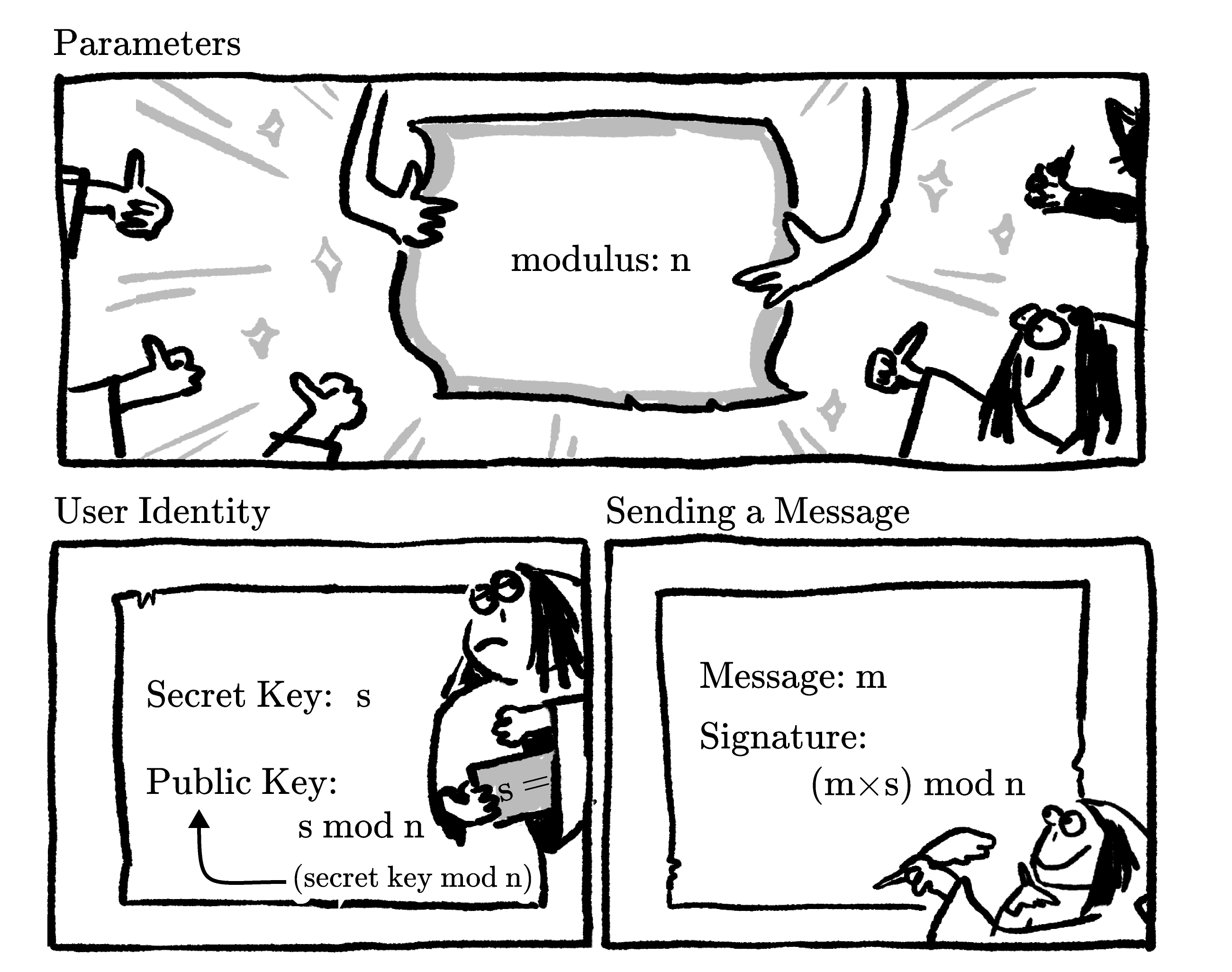The last step of the system is that we need to be able to verify each $\tt signature$, confirming that it used the correct $\tt secretKey$ when it was created. What needs to be true of $(m \times s)\bmod{n}$ in order for it to be possible to verify a $\tt signature$ in this new scheme using only public information?

# Cryptographic Signatures

For our system to work, we also need to be able to verify each $\tt signature$. Helping us achieve this is the fact that even though the modular product hides the factors going into it, associativity and commutativity still apply.

In simpler terms, this means that the order in which you multiply the numbers and apply $\bmod{\>n}$ doesn't matter. You'll always get the same result after applying a final $\bmod{\>n}$ at the end.

Consider an example where $m = 569, s = \num{1187},$ and $n=447$. Whether we apply $\bmod{}$ at every step, or only apply $\bmod{}$ after multiplying the message and secret key, we'll get the same result:

 Apply $\bmod{}$ at every step Apply $\bmod{}$ only at the end $569\bmod{447} = 122$ $569 \times \num{1187} = \num{675403}$ $\num{1187}\bmod{447} = 293$ $\num{675403}\bmod{447} = 433$ $122 \times 293 = \num{35746}$ $\num{35746}\bmod{447} = 433$

It's not crucial for you to understand why this is the case, but if you're curious, it's because whether you apply $\bmod{\>n}$ before or after multiplying, it still has the effect of removing multiples of $n$ from the product. Any multiples of $n$ that make it through to the end will be removed by the final $\bmod{\>n}$, leading to the same result:

# Cryptographic Signatures

Since the order of modular products doesn't change the final outcome, we can add the final step of verification to our system. Anyone can verify a $\tt signature$ by confirming that it equals $(\texttt{publicKey} \times m)\bmod{n}$. This works because \begin{aligned} \texttt{signature} &= (\texttt{publicKey} \times m)\bmod{n} \\ (m\times s)\bmod{n} &= \big((s\bmod{n}) \times m\big)\bmod{n}. \end{aligned} And whether you take the modular product of $m$ and $s$ or the modular product of $(s\bmod{n})$ and $m,$ you'll get the same result.

Here's an overview of all the steps: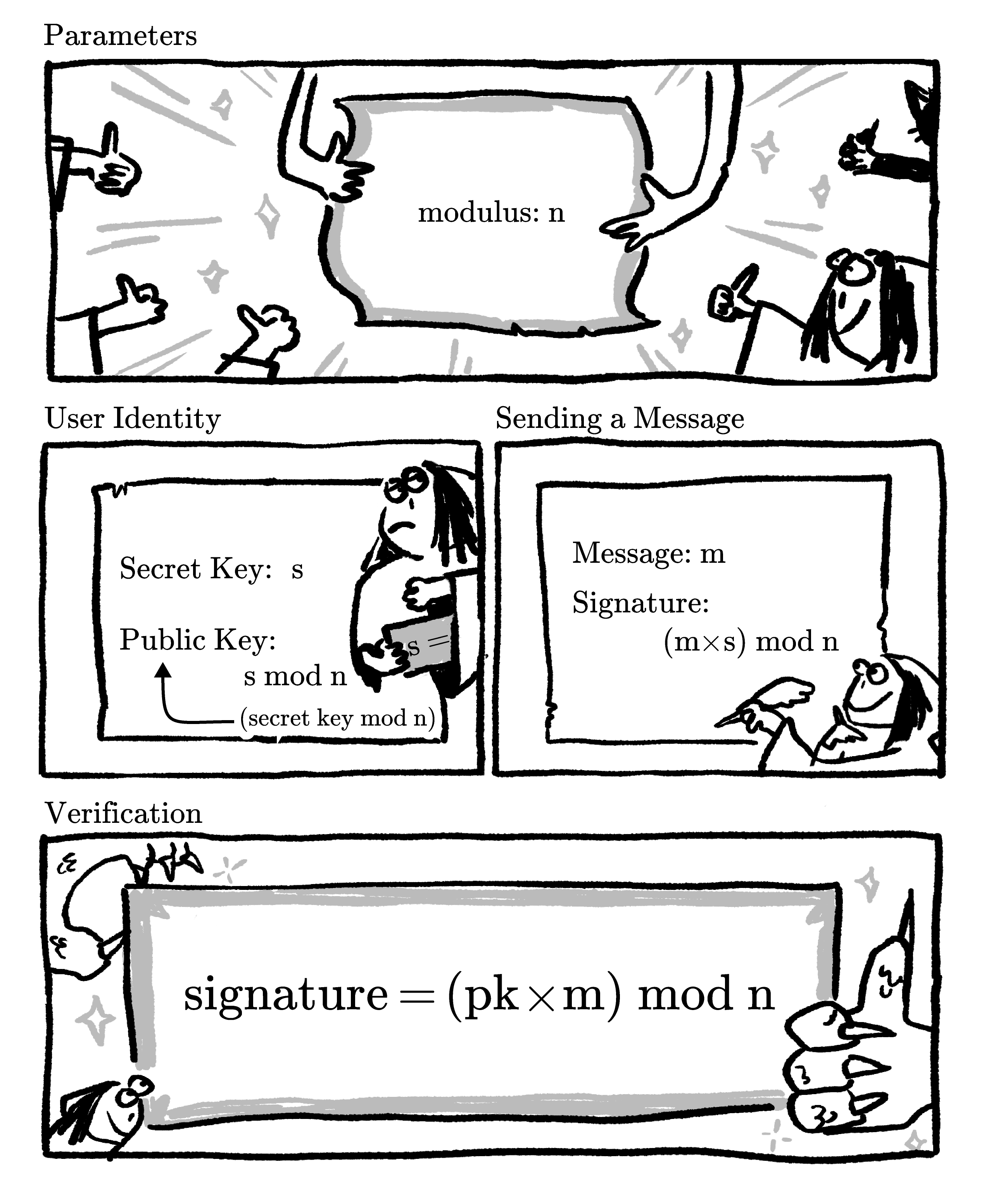With this implementation of $\bmod$ into our system, are all the steps secure?

# Cryptographic Signatures

For the purposes of multiplication in $\bmod{\>n}$, knowing the remainder of the $\tt secretKey$ is equivalent to knowing the $\tt secretKey$ itself, so a $\tt publicKey$ of $s \bmod{n}$ isn't secure.

Fortunately, the fix isn't far off. We just saw that even if we know one number of a product, it's still hard to find the other one: ${(m \times s) \bmod{n}}$ hides $s$ even if we know $m$.

With this in mind, we can change our scheme just a little bit:

Suppose everybody agrees on a common number $g$. They still pick a $\tt secretKey$ $s$ as before, but now they share $(g \times s) \bmod{n}$ as their $\tt publicKey$. The $\tt signature$ for a $\tt message$ $m$ is still $(m \times s)\bmod{n}$, but now the verification happens by confirming that $(\texttt{publicKey} \times m)\bmod{n} = (\texttt{signature} \times g)\bmod{n}.$ These will be equal for a valid $\tt signature$ because both sides of the equation contain only $g, s,$ and $m$ as factors.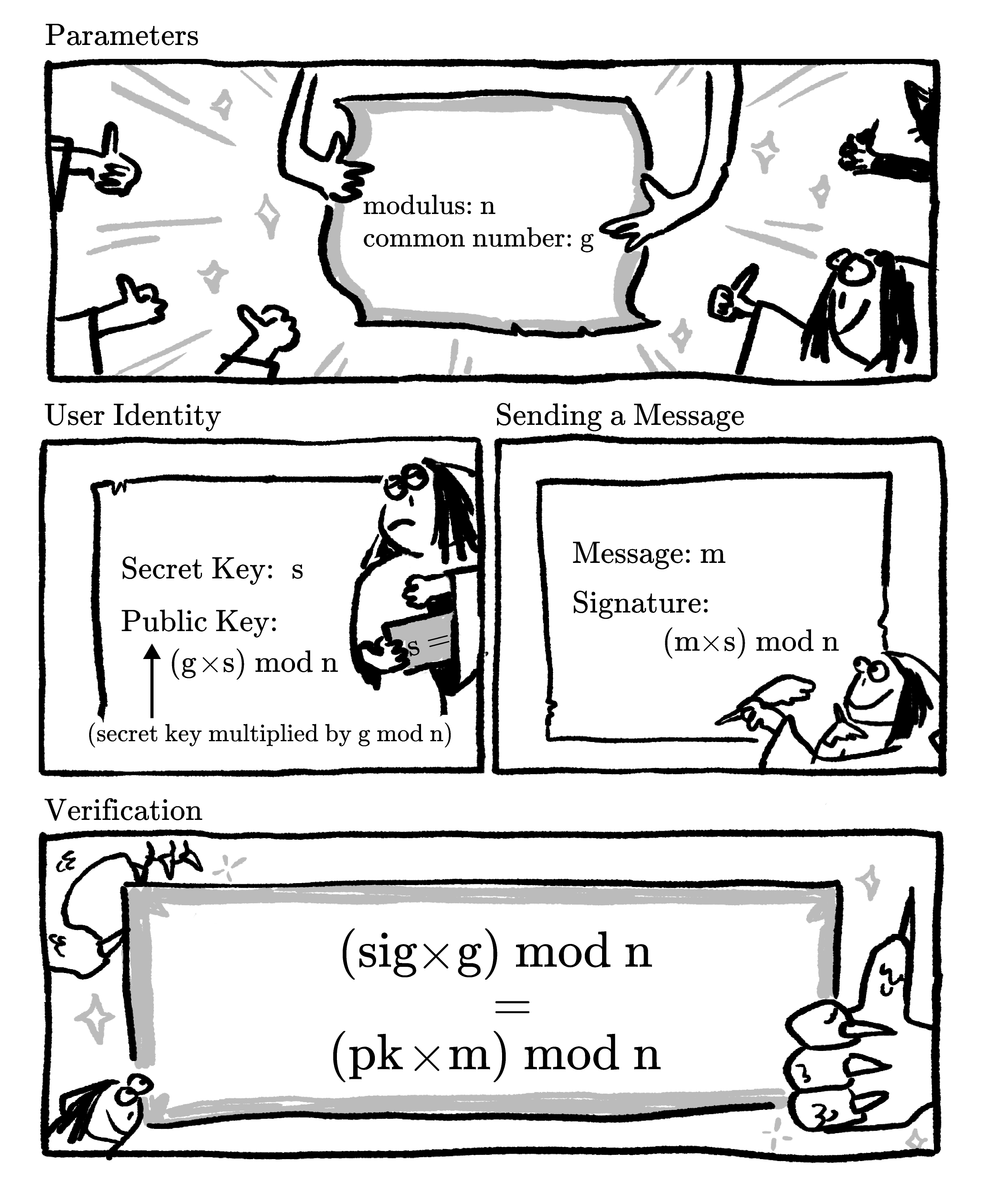Suppose we use this system with $n = 179$ and $g = 59.$ If you receive the $\tt message$ $101$ from Alice, whose $\tt publicKey$ is $24$, and the included $\tt signature$ is $123,$ was the $\tt message$ really sent by Alice? Assume Alice is the only person with access to her $\tt secretKey$.

The calculator below (recovered from Cryptonia) is programmed with the modular arithmetic of the DragonBucks system. You can use it to help to answer the question:

# Cryptographic Signatures

With this new system, the Cryptonians have successfully divorced their DragonBucks scheme from spellcasting, and can open it up to everyone regardless of their magical abilities. Their modular products scheme has three key features:

1. Everyone has an identity that no one else can fake.
2. Everyone can sign transactions.
3. Everyone can verify that transactions are valid.

A serviceable mathematician herself, the dragon is satisfied with the security of mathematically signed DragonBucks and is happy to process them, allowing the magically challenged newcomers to fully participate in the Cryptonian economy.

To facilitate the spread of the system, the Cryptonian scholars made calculators that could quickly calculate large modular products and distributed these calculators among the townsfolk.

If you're a number theory wizard yourself, you've probably noticed a problem with the security of DragonBucks. Don't worry, this will be addressed later in the course.

# Cryptographic Signatures

×